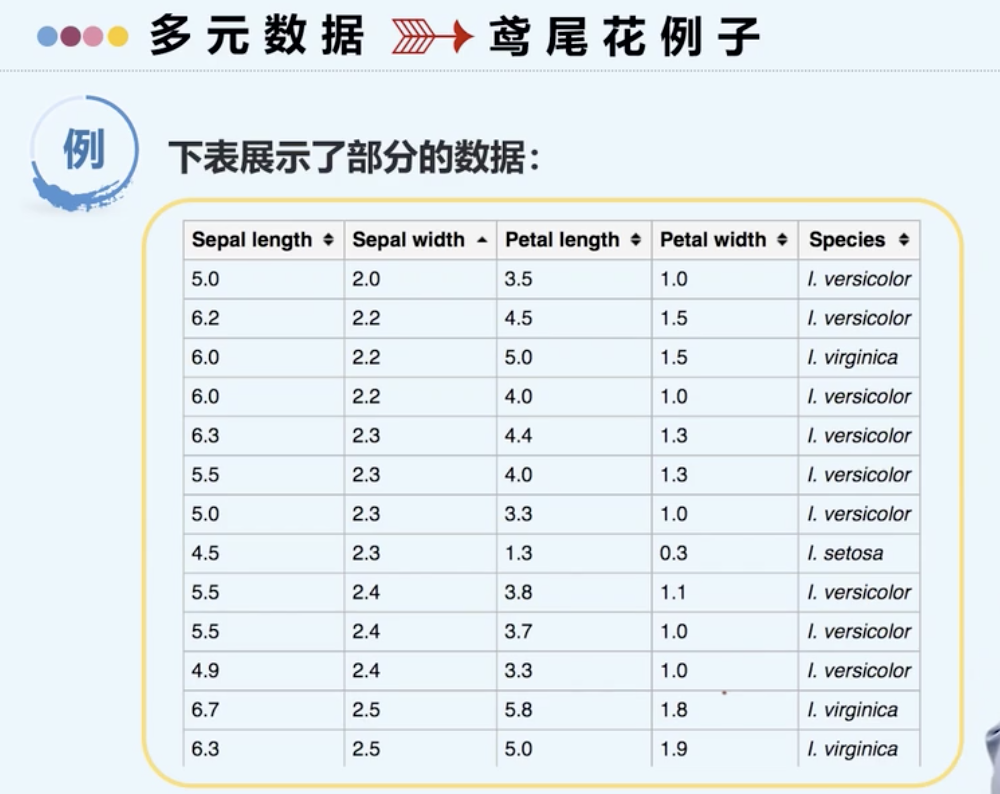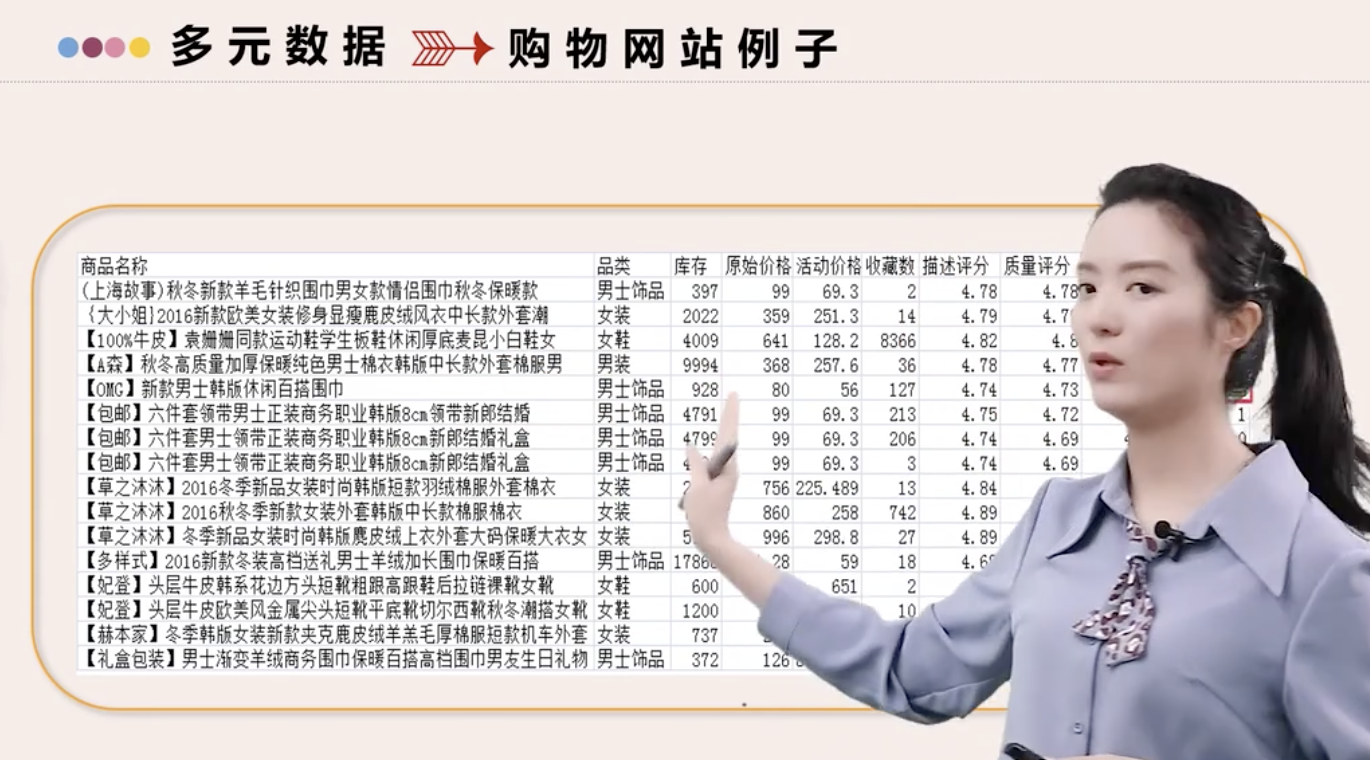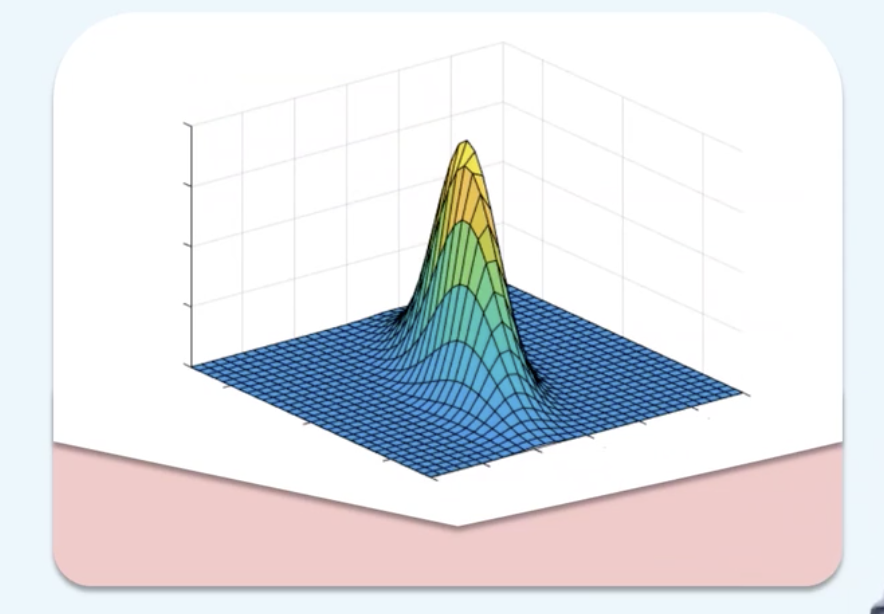• 多元统计分析课件多元统计分析课件多元统计分析课件
• 多元统计经典教科书。多元统计分析是从经典统计学中发展起来的一个分支，是一种综合分析方法，它能够在多个对象和多个指标互相关联的情况下分析它们的统计规律
• b站看【厦门大学MOOC】多元统计分析，因为老师很好看。 参考： 【厦门大学MOOC】多元统计分析 https://www.bilibili.com/video/BV1v7411E7PB 课程大纲： 一、多元统计分析概述 二、多元数据的描述与展示 三、多元...
〇、前情提要
b站看【厦门大学MOOC】多元统计分析，因为老师很好看。
参考：

【厦门大学MOOC】多元统计分析
https://www.bilibili.com/video/BV1v7411E7PB

课程大纲：
一、多元统计分析概述
二、多元数据的描述与展示
三、多元正态分布
四、均值向量的检验
五、判别分析和分类分析
六、主成分分析
七、因子分析
八、聚类分析

一、多元统计分析概述1.1 多元分析的定义
多元统计分析是什么？
多元统计分析定义多元数据例子
鸢尾花例子行：样本     列：信息维度->研究变量之间的相关性、做回归
购物网站例子-> 维度之间的关系
-> 业务问题

1.2 多元分析的方法简介数据描述
多元数据特征和可视化、多元正态分布

怎么从特征上面去描述：多元数据波动性、平均情况、变量与变量之间的相关性
怎么用图形表示
多元正态分布情况

第二章、第三章
统计推断
多元数据的统计检验

假设检验（数理统计 一个变量时均值的检验、两样本t检验）

第四章
经典降维
简化数据结构

具体怎么做

第六章、第七章
目标归类
根据数据特征构造归类模式

特征 聚类问题
标签 分类 判别分析问题

第八章、第五章数据描述
将从四个部分来讲（第二章）数据都是有分布的，多元正态（第三章）
二元正态顾客满意度评分
平均、波动性、相关性微博活跃程度统计推断
μ=μ0的推广（第四章）经典降维不是所有的信息都有用
用少数代替多数主成分分析
样本/个体之间差异 最大化方差
因子分析
综合指标/公共因子 变量与变量之间的相关性 有公共因素
数据减肥目标归类
对新的样本分类多种信息维度分类监督学习-分类问题
分类问题第五章无监督学习-聚类问题
聚类问题第八章1.3 多元分析的应用领域
用统计学原理，研究各种感兴趣领域的知识。不同行业的应用
聚类 分类 判别问题
市场营销银行业金融行业医疗行业分子生物学天文学法务会计如何使数据驱动价值
有原始数据
->直观有效信息（二三章 可视化 波动性 平均情况 分布性）
->提取有用的知识（统计推断 显著）
->统计建模（回归 分类）展开全文• 陈峰的医用多元统计 逻辑清晰 也不繁琐 还有大量的示例
• 多元统计作业进行多总体的均值方差检验 形象分析，学习多元统计的知识，这是大数据的预备知识
•统计学
• 多元统计分析概述目录一、引言…………………………………………………………3二、多元统计分析方法的研究对象和主要内容……………31.多元统计分析方法的研究对象…………………………32.多元统计分析方法的主要...
多元统计分析概述目录一、引言…………………………………………………………3二、多元统计分析方法的研究对象和主要内容……………31.多元统计分析方法的研究对象…………………………32.多元统计分析方法的主要内容…………………………3三、各种多元统计分析方法…………………………………31.回归分析…………………………………………………32.判别分析………………………………………………63.聚类分析………………………………………………84.主成分分析………………………………………………105.因子分析………………………………………………106.对应分析方法…………………………………………117.典型相关分析…………………………………………11四、多元统计分析方法的一般步骤……………………………12五、多元统计分析方法在各个自然领域中的应用……………12六、总结…………………………………………………………13参考文献…………………………………………………………14谢辞………………………………………………………………15
展开全文• 介绍多元统计的基础概念。
文章目录一、多元统计基础1.基本定义与样本数据阵2.随机向量的分布3.随机向量数字特征4.随机向量数字特征的性质总结回顾
一、多元统计基础
1.基本定义与样本数据阵
多元统计与一元统计的最大不同，就在于一元统计的样本全部来自一元总体，而多元总体的样本来自多元总体，用多个随机变量刻画它的多个维度。比如，要获得人的身高分布情况，只要对一个人群中体的样本测量身高，这是一个一元随机变量；而要获得人的身高、体重分布情况，每一个样本就要测量身高、体重，这样组成一个二元随机向量。
很显然，随机向量中的每一个分量都是随机变量，那么对多元总体进行分析时，我们能否分开每一个随机变量分量呢？这是不合理的，因为不同的随机变量之间很可能存在着关联。比如身高、体重显然是存在的关联的，所以往往用BMI衡量人的身体质量情况，如果分开研究，就会打破它们之间的联系，失去一部分信息。
因此，多元统计其实是对多维随机向量的研究，而不是对分开的随机变量的研究。为了描述随机向量的性质，我们需要使用一定的概念来描述。要注意的是，以下出现的向量一般都是列向量。
一个总体中每一个个体具有$p$个属性，它们或存在关联或不存在关联，这$p$个属性分别用$p$个随机变量总体$X_1,\cdots,X_p$来表示。从总体$X=(X_1,\cdots,X_p)'$中抽取$n$个样本，每一个样本记作$X_{(1)},\cdots,X_{(n)}$（一般在下标加括号与属性总体区分），这样，每一个样本还可以表示成
$X_{(i)}=(X_{i1},X_{i2},\cdots,X_{ip})',$
将$n$个样本纵向排列，就得到一个$n\times p$矩阵，称为样本数据阵，如下：
$X=\begin{bmatrix} x_{11}&x_{12}&\cdots&x_{1p}\\ x_{21}&x_{22}&\cdots&x_{2p}\\ \vdots&\vdots&&\vdots\\ x_{n1}&x_{n2}&\cdots&x_{np} \end{bmatrix}=\begin{bmatrix} X_{(1)}'\\X_{(2)}'\\\vdots\\X_{(n)}' \end{bmatrix}\stackrel {\rm def}=(\mathcal X_1,\cdots,\mathcal X_p) .$
样本数据阵的相关概念如下有：

第$i$行$X_{(i)}'$：代表第$i$个$p$维样本，具有两重性。在观测前，它是$p$维随机向量；在观测后，它是$p$维向量。
第$j$列$\mathcal X_j$：代表第$j$个属性的$n$个观测值，相当于将样本的每个属性分开研究，每一个构成一个样本容量为$n$的样本。

2.随机向量的分布
对$n$个样本进行研究，用到数理统计的知识，多元情况下也不例外。在一元的情形，数理统计是依赖于概率论，尤其是其中的几个重要分布；因此在进行多元统计之前，有必要对随机向量的分布进行讨论。随机向量的分布，指的是联合分布、边缘分布、条件分布等。
$p$维随机向量$X$的联合分布是一个$p$元函数
$F(x_1,\cdots,x_p)={\rm P}(X_1\le x_1,\cdots,X_p\le x_p).$
类似一元的情况，如果有一个$p$元非负函数，使得对一切$(x_1,\cdots,x_p)\in \R^p$，都有
$F(x_1,\cdots,x_p)=\int_{-\infty}^{x_1}\cdots\int_{-\infty}^{x_p}p(s_1,\cdots,x_p){\rm d}(s_1,\cdots,s_p),$
就称$p(x_1,\cdots,x_p)$是$X$的联合概率密度。
如果我们只考虑随机变量的部分分量$(X_{i_1},\cdots,X_{i_m}),1\le m的分布，则部分分量的联合分布，称为$X$的边缘分布。要求某几个分量的边缘分布，只需要将联合分布$F(x_1,\cdots,x_p)$中不关心的那部分分量值取为$+\infty$，剩下的就是边缘分布。

如二元总体$X=(X_1,X_2)$的联合分布是$F(x_1,x_2)$，则$X_1$的边缘分布是$F(x_1,\infty)$，$X_2$的边缘分布是$F(\infty,x_2)$。
如果已知总体联合密度，要求边缘密度，则将无关部分进行积分。如二元总体$X=(X_1,X_2)$的联合密度是$p(x_1,x_2)$，则$X_1$的边缘密度就是$\int_{-\infty}^\infty p(x_1,x_2){\rm d}x_2$，$X_2$的边缘密度就是$\int_{-\infty}^\infty p(x_1,x_2){\rm d}x_1$。

条件分布指的是给定一部分分量时，另一部分分量的分布。假如$X=(X^{(1)},X^{(2)})$，这里$X^{(1)}$是$r$维随机向量，$X^{(2)}$是$p-r$维随机向量，则给定$X^{(2)}$时$X^{(1)}$的条件分布是$F(X^{(1)}|X^{(2)})$。

如果$X$的联合密度是$p(x^{(1)},x^{(2)})$，则条件密度为
$p(x^{(1)}|x^{(2)})=\frac{p(x^{(1)},x^{(2)})}{p(x^{(2)})}.$

定义条件分布后，可以定义随机向量分量的独立性。如果$F(x_1,\cdots,x_p)=F_1(x_1)\cdots F_p(x_p)$，这里$F_1(x),\cdots,F_p(x)$是$X_1,\cdots,X_p$的边缘分布，则称$X_1,\cdots,X_p$相互独立。同理，如果$f(x_1,\cdots,x_p)=f_1(x_1)\cdots f_p(x_p)$，这里$f_1(x),\cdots, f_p(x)$是$X_1,\cdots,X_p$的边缘密度，也称$X_1,\cdots,X_p$相互独立。
以上分布的定义方式，均与一元非常类似，只要区分联合、边缘的区别即可。
3.随机向量数字特征
在一元总体中，我们定义过均值、方差等数字特征，在多元中也可以类似定义一系列数字特征，用来刻画分布的部分性质。不同的是，在多元统计中，我们还需要考虑一个随机向量内部的结构。
对于$X=(X_1,\cdots,X_p)$，如果对每个分量$X_i$都有${\rm E}X_i=\mu_i$存在，则定义随机向量的均值向量为
${\rm E}(X)=\begin{bmatrix} {\rm E}(X_1)\\{\rm E}(X_2)\\\vdots\\{\rm E}(X_p) \end{bmatrix}=\begin{bmatrix} \mu_1\\\mu_2\\\vdots\\\mu_p \end{bmatrix}.$
多元向量中，每一个分量的方差还有两个分量之间的协方差，可以用一个协方差矩阵来囊括。如果对任何$i,j$，都有${\rm Cov}(X_i,X_j)={\rm E}(X_i-\mu_i)(X_j-\mu_j)=\sigma_{ij}$存在，则定义协方差阵为
${\rm D}(X)=\begin{bmatrix} \sigma_{11}&\sigma_{12}&\cdots&\sigma_{1p}\\ \sigma_{21}&\sigma_{22}&\cdots&\sigma_{2p}\\ \vdots&\vdots&&\vdots\\ \sigma_{p1}&\sigma_{p2}&\cdots&\sigma_{pp} \end{bmatrix}=(\sigma_{ij})_{p\times p}\stackrel {\rm def}=\Sigma.$

可以注意到，成立以下等式：
${\rm D}(X)={\rm E}[(X-{\rm E}(X))(X-{\rm E}(X))'].$

类似一元随机变量中由协方差定义相关系数的方式，我们可以定义相关系数阵。如果令$r_{ij}=r_{X_i,X_j}={\rm Cov}(X_i,X_j)/\sqrt{{\rm D}(X_i){\rm D}(X_j)}=\sigma_{ij}/\sqrt{\sigma_{ii}\sigma_{jj}}$，那么定义相关系数阵为
$R=\begin{bmatrix} r_{11}&r_{12}&\cdots&r_{1p}\\ r_{21}&r_{22}&\cdots&r_{2p}\\ \vdots&\vdots&&\vdots\\ r_{p1}&r_{p2}&\cdots &r_{pp} \end{bmatrix}=(r_{ij})_{p\times p}.$

如果记$V^{1/2}={\rm diag}(\sqrt{\sigma_{11}},\cdots,\sqrt{\sigma_{pp}})$为$X$的标准差矩阵，就成立以下等式：
$\Sigma=V^{1/2}RV^{1/2},\quad R=V^{-1/2}\Sigma V^{-1/2}.$

对于两个总体$X,Y$，分别是$p$维和$q$维向量，其协方差阵为${\rm COV}(X,Y)={\rm E}[(X-{\rm E}(X))(Y-{\rm E}(Y))']$，如果${\rm COV}(X,Y)=O_{p\times q}$，则称总体$X,Y$不相关。

可以注意到，成立以下等式：
${\rm COV}(X,Y)=[{\rm COV}(Y,X)]'.$

4.随机向量数字特征的性质
首先是随机向量的运算性质：
$\begin{array}{l} {\rm E}(AXB)=A{\rm E}(X)B,\\ {\rm D}(AX)=A{\rm D}(X)A',\\ {\rm COV}(AX,BY)=A{\rm COV}(X,Y)B'. \end{array}$
这里$A,B$是常数矩阵（满足运算要求），$X,Y$是随机向量。这些运算性质需要牢记，有很广泛的应用。
类比随机变量独立、不相关的关系，我们得出${\rm COV}(X,Y)=O_{p\times q}$是$X,Y$独立的必要不充分条件，即不相关不一定独立，但独立一定不相关。
关于随机向量的自协方差矩阵$\Sigma$，又有一些独特的性质：

对于任何随机向量$X$，其自协方差矩阵$\Sigma$是非负定对角阵。
$\Sigma=L^2$，这里$L$为非负定矩阵，当$\Sigma>0$时称为$\Sigma$的平方根矩阵。只要注意到非负定对角阵可正交对角化即可。
如果$\Sigma$的特征值是$\lambda_1,\cdots,\lambda_p$，则由正定性，所有特征值$\ge 0$，那么$\Sigma=\Gamma(\lambda_1,\cdots,\lambda_p)\Gamma'$，这里$\Gamma$是正交矩阵。所以令$A=\Gamma(\sqrt\lambda_1,\cdots,\sqrt\lambda_p)$，则$\Sigma=AA'$。

总结回顾

样本数据阵，是将$n$个$p$维向量（即样本）按列排构成的矩阵。矩阵中每一列代表一个样本的观测值，每一行代表一个属性维度。

随机向量具有联合分布、边缘分布和条件分布，如果是连续型随机向量，则还有联合密度、边缘密度和条件密度。

由条件分布、条件密度刻画了随机向量分量间的独立性，当联合分布（密度）可拆分为边缘分布（密度）的乘积时，代表分量独立。

随机向量具有均值向量${\rm E}(X)$、自协方差矩阵${\rm D}(X)$、自相关矩阵$R$、标准差对角阵$V^{1/2}$等数字特征，刻画两个随机向量的相关程度用协方差矩阵。如果协方差矩阵为0矩阵，则两个随机向量不相关。

随机向量的数字特征之间存在以下联系：
${\rm D}(X)={\rm E}[(X-{\rm E}(X))(X-{\rm E}(X))'],\\ {\rm D}(X)=V^{1/2}RV^{1/2},R=V^{-1/2}{\rm D}(X)V^{-1/2},\\ {\rm COV}(X,Y)=[{\rm COV}(Y,X)]'.$

有以下计算性质是需要记忆的：
${\rm E}(AXB)=A{\rm E}(X)B,\\ {\rm D}(AX)=A{\rm D}(X)A',\\ {\rm COV}(AX,BY)=A{\rm COV}(X,Y)B'.$

随机向量的协方差矩阵$\Sigma$是非负定对称阵，可以正交分解为$\Gamma \Lambda\Gamma'$，这里$\Gamma$是正交矩阵，$\Lambda$是特征值对角阵。如果$A=\Gamma \Lambda^{1/2}$，则$\Sigma=AA'$；如果$L=\Gamma \Lambda^{1/2}\Gamma'$，则$\Sigma=L^2$，当$\Sigma>0$时$L$也是正定的，称为$\Sigma$的平方根矩阵。


展开全文• 多元统计分析重点宿舍版第一讲：多元统计方法及应用；多元统计方法分类(按变量、模型、因变量等)多元统计分析应用选择题：①数据或结构性简化运用的方法有：多元回归分析，聚类分析，主成分分析，因子分析②分类和...
多元统计分析重点宿舍版第一讲：多元统计方法及应用；多元统计方法分类(按变量、模型、因变量等)多元统计分析应用选择题：①数据或结构性简化运用的方法有：多元回归分析，聚类分析，主成分分析，因子分析②分类和组合运用的方法有：判别分析，聚类分析，主成分分析③变量之间的相关关系运用的方法有：多元回归，主成分分析，因子分析，④预测与决策运用的方法有：多元回归，判别分析，聚类分析因果模型(因变量数)：多元回归，判别分析⑤横贯数据：｛相依模型(变量测度)：因子分析，聚类分析多元统计分析方法选择题：①多元统计方法的分类：1)按测量数据的来源分为：横贯数据(同一时间不同案例的观测数据)，纵观数据(同样案例在不同时间的多次观测数据)2)按变量的测度等级(数据类型)分为：类别(非测量型)变量，数值型(测量型)变量3)按分析模型的属性分为：因果模型，相依模型4)按模型中因变量的数量分为：单因变量模型，多因变量模型，多层因果模型第二讲：计算均值、协差阵、相关阵；相互独立性第三讲：主成分定义、应用及基本思想，主成分性质，主成分分析步骤主成分定义：何谓主成分分析就是将原来的多个指标(变量)线性组合成几个新的相互无关的综合指标(主成分)，并使新的综合指标尽可能多地反映原来的指标信息。主成分分析的应用：(1)数据的压缩、结构的简化；(2)样品的综合评价，排序主成分分析概述——思想：①(1)把给定的一组变量X1,X2,…XP,通过线性变换，转换为一组不相关的变量Y1，Y2，…YP。(2)在这种变换中，保持变量的总方差(X1，X2，…Xp的方差之和)不变，同时，使Y1具有最大方差，称为第一主成分；Y2具有次大方差，称为第二主成分。依次类推，原来有P个变量，就可以转换出P个主成分(3)在实际应用中，为了简化问题，通常找能够反映原来P个变量的绝大部分
展开全文• 关注公众号，更多资源分享回复关键词：多元统计分析即可获取更多详细其他章节答案《多元统计分析》课后答案||何晓群版(第二章)《多元统计分析》第一章课后答案(何晓群编第五版)《多元统计分析》课后答案||何晓群版(2...
• 告诉你如何使用R统计软件实现一些简单的多元统计分析，也意味着我们将... 这本小册子假设读者已经拥有一定的多元统计分析的基础知识，并且本册的首要任务并不是解释多元统计分析，而是解释如何使用R实现多元统计分析。R语言
• 多元统计分析教程 介绍常用的各种多元统计分析方法,包括判别分析、聚类分析、主成分分析、相关分析和因子分析等……
• 多元统计分析的教材，很清晰 应用多元统计分析 高惠璇数学
• 对我国主要城市的气温进行多元统计分析，采用spss软件进行聚类分析，因子分析，相关分析，得出结论用于指导我国各城市的生产生活实践。
• 多元统计分析的聚类分析课件，利用多元统计分析可以实现聚类分析聚类分析
• 多元统计分析习题及解答多元统计分析习题及解答第一章 绪论填空题1．多元分析研究的是______________的统计总体。2．多元统计中常用的统计量有：________、_________、__________和__________。3．协方差和相关系数...
• 非常实用的多元统计学习课本，本书可以自学，也可以作为学习多元统计的参考资料，里面叙述非常详细...